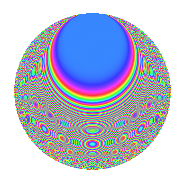# Properties

 Label 320.6.nLevel 320 Weight 6 Character orbit n Rep. character $$\chi_{320}(63,\cdot)$$ Character field $$\Q(\zeta_{4})$$ Dimension 116 Sturm bound 288

# Related objects

## Defining parameters

 Level: $$N$$ $$=$$ $$320 = 2^{6} \cdot 5$$ Weight: $$k$$ $$=$$ $$6$$ Character orbit: $$[\chi]$$ $$=$$ 320.n (of order $$4$$ and degree $$2$$) Character conductor: $$\operatorname{cond}(\chi)$$ $$=$$ $$20$$ Character field: $$\Q(i)$$ Sturm bound: $$288$$

## Dimensions

The following table gives the dimensions of various subspaces of $$M_{6}(320, [\chi])$$.

Total New Old
Modular forms 504 124 380
Cusp forms 456 116 340
Eisenstein series 48 8 40

## Trace form

 $$116q + 4q^{5} + O(q^{10})$$ $$116q + 4q^{5} + 4q^{13} - 812q^{17} + 8q^{21} + 6228q^{25} + 968q^{33} + 4q^{37} - 8q^{41} - 12496q^{45} - 77708q^{53} - 976q^{57} - 96152q^{61} + 35364q^{65} + 10068q^{73} - 308232q^{77} - 371772q^{81} + 31636q^{85} + 364744q^{93} - 103548q^{97} + O(q^{100})$$

## Decomposition of $$S_{6}^{\mathrm{new}}(320, [\chi])$$ into newform subspaces

The newforms in this space have not yet been added to the LMFDB.

## Decomposition of $$S_{6}^{\mathrm{old}}(320, [\chi])$$ into lower level spaces

$$S_{6}^{\mathrm{old}}(320, [\chi]) \cong$$ $$S_{6}^{\mathrm{new}}(20, [\chi])$$$$^{\oplus 5}$$$$\oplus$$$$S_{6}^{\mathrm{new}}(80, [\chi])$$$$^{\oplus 3}$$$$\oplus$$$$S_{6}^{\mathrm{new}}(160, [\chi])$$$$^{\oplus 2}$$

## Hecke characteristic polynomials

There are no characteristic polynomials of Hecke operators in the database